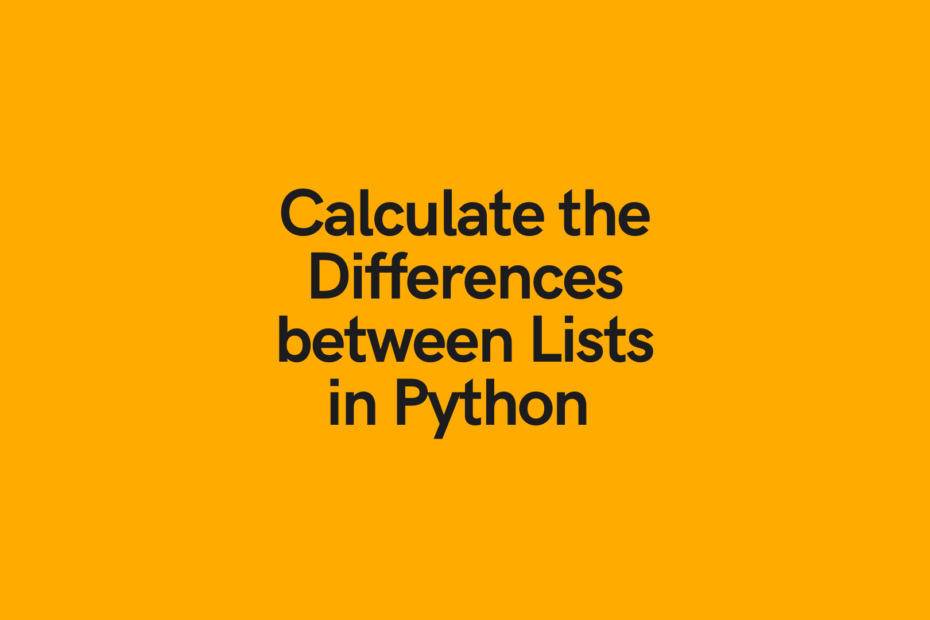# Python List Difference: Find the Difference between 2 Python ListsIn this post, you’ll learn how to find the Python list difference, meaning what items are different between two lists.

In particular, you’ll learn: how to find the difference between lists when order and duplicates matters, when they don’t matter, and how to find the symmetrical difference between two lists.

The Short Answer: Use Set Subtraction

``````list1 = [1,2,3,4,5,6]
list2 = [2,4,5,7]

difference = list(set(list1) - set(list2))
print(difference)

# Returns [1, 3, 6]``````

## What is Python List Difference?

Python list difference refers to finding the items that exist in one list, but not in the other. There are two main things to understand here:

1. The comparison ordering matters: if you want to find the items in one list but not in the other, you need to use that are your comparator
2. Whether repetition matters: since lists can contain duplicate values, some methods will work better for this

### Comparison Ordering

Say we have two different lists:

``````list1 = [1,2,3,4,5,6]
list2 = [2,4,5,7]
``````

If we were to calculate the difference between list1 and list2, we would find that list1 contains `[1, 3, 6]`, while list2 doesn’t.

If we were to calculate the difference between list2 and list1, we would find that list2 contains ``, while list1 doesn’t.

If you’re looking to find the list of items that exist in either list but not both, check out the section on finding Python symmetrical difference.

### Duplicates in Lists

Now, to understand better, let’s look at three different lists:

``````list1 = [1,2,3,4,5,6]
list1b = [1,2,3,3,4,5,6]
list2 = [2,4,5,7]``````

We have lists `list1` and `list2` which contain only unique items. However, we also have `list1b` that contains two 3’s. Were we to calculate a duplicate list difference:

• Between `list1` and `list2`, we would return `[1,3,6]`.
• However, were we do to the same between `list1b` and `list2`, we would return `[1,3,3,6]`.

Now, were we to calculate non-repetitve list differences, both differences would simply be `[1,3,6]`.

## Calculate List Difference with Duplicate Items with a For Loop

In order to calculate a repetitive list difference in Python, we can use a for-loop. For a refresher on for-loops, check out my post here and my video tutorial here:

Let’s see how we could calculate the repetitive difference using a for loop:

``````list1 = [1,2,3,3,4,5,6]
list2 = [2,4,5,7]

difference = list()

for item in list1:
if item not in list2:
difference.append(item)

print(difference)

# Returns: [1, 3, 3, 6]``````

We can also run the reverse of this to calculate the difference between `list2` and `list1`:

``````list1 = [1,2,3,3,4,5,6]
list2 = [2,4,5,7]

difference = list()

for item in list2:
if item not in list1:
difference.append(item)

print(difference)

#Returns: ``````

## Calculate List Difference with a List Comprehension

For loops can be a bit cumbersome to write, especially given the need to declare an empty list first. We can accomplish the same task by using a list comprehension.

For a refresher on list comprehensions, check out my post here and my video tutorial here:

Now, let’s see how we can use a list comprehension to find the differences between two lists:

``````list1 = [1,2,3,4,5,6]
list2 = [2,4,5,7]

list1diff2 = [item for item in list1 if item not in list2]
list2diff1 = [item for item in list2 if item not in list1]

print('list1diff2 is: ', list1diff2)
print('list2diff1 is: ', list2diff1)

# Returns:
# list1diff2 is:  [1, 3, 6]
# list2diff1 is:  ``````

While you sacrifice a little bit of readability, you do gain the freedom to write this entire comprehension on a single line of code!

## Python List Difference with Set Subtraction

Finding the list difference between two lists if you don’t need to worry about repetition is a lot faster!

What we’ll do, is:

1. Convert both lists to sets,
2. Find the difference between both sets, and
3. Convert the resulting set to a list

Let’s see how we can accomplish this:

``````list1 = [1,2,3,4,5,6]
list2 = [2,4,5,7]

difference = list(set(list1) - set(list2))
print(difference)

# Returns: [1,3,6]``````

The benefit of this approach is speed! Working with sets is much more efficient than iterating over items in lists. The trade-off, as mentioned before, is that repeated items will only show up one time.

## Calculate List Difference with the Set .difference Method

Similar to the example above, we can use the `.difference()` method to calculate the difference between two lists.

In this approach, we will:

1. Convert the comparison list to a set,
2. Apply the `.difference()` method to it and pass in the other list
3. Convert it back to a list

Let’s see what this looks like in practice:

``````list1 = [1,2,3,4,5,6]
list2 = [2,4,5,7]

difference = list(set(list1).difference(list2))
print(difference)

# Returns: [1,3,6]``````

Check out some other Python tutorials on datagy, including our complete guide to styling Pandas and our comprehensive overview of Pivot Tables in Pandas!

## How to calculate Python Symmetrical List Difference?

The symmetrical difference in Python is a list of items that exist in either list, but not both lists. Recall that with all the methods above, we’re really saying: “these items exist in the comparison list, but not in the other”.

With symetrical difference, we’re saying: “these items exist in either list, but not in both”.

In order to accomplish this, we will rely on the `.symmetric_difference()` method.

``````list1 = [1,2,3,4,5,6]
list2 = [2,4,5,7]

symmetrical_difference = list(set(list1).symmetric_difference(set(list2)))
print(symmetrical_difference)

# Returns: [1,3,6,7]``````

The above code may be a bit complex to read, so let’s break it into a few more steps:

``````list1 = [1,2,3,4,5,6]
list2 = [2,4,5,7]

set1 = set(list1)
# {1,2,3,4,5,6}

set2 = set(list2)
# {2,4,5,7}

set_difference = set1.symmetric_difference(set2)
# {1,3,6,7}

list_difference = list(set_difference)
print(list_difference)
# Returns: [1,3,6,7]``````

This accomplishes the same thing, but may be a bit easier to follow!

## Conclusion

In this post, you learned how to calculate the Python list difference. This means you learned how to find items that exist in one list but not in the other. You learned how to do this using for-loops, list comprehensions, and set differences.

You also learned how to calculate the symmetric difference between two lists, meaning finding items that exist in either list, but not in both.